• Slides: 23Anatomy of a linked list • A linked list consists of: – A sequence of nodes my. List a b c Each node contains a value and a link (pointer or reference) to some other node The last node contains a null link The list may have a header d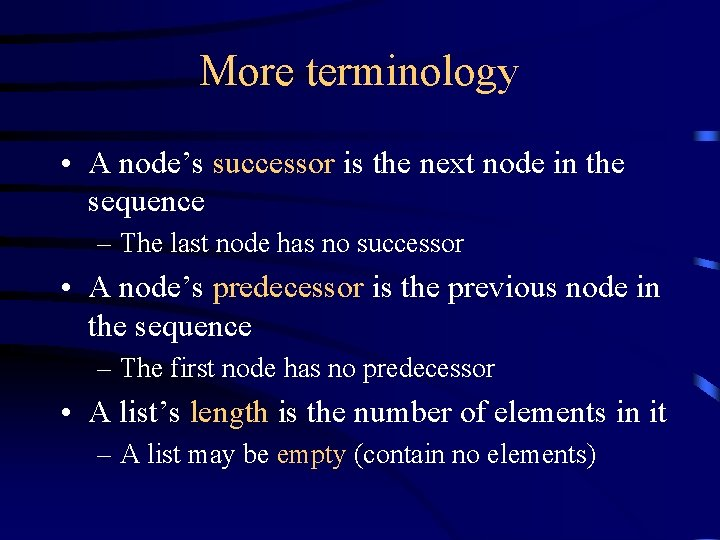More terminology • A node’s successor is the next node in the sequence – The last node has no successor • A node’s predecessor is the previous node in the sequence – The first node has no predecessor • A list’s length is the number of elements in it – A list may be empty (contain no elements)Singly-linked lists • Here is a singly-linked list (SLL): my. List a b c d • Each node contains a value and a link to its successor (the last node has no successor) • The header points to the first node in the list (or contains the null link if the list is empty)Singly-linked lists in Java (p. 69) public class SLL { private SLLNode first; public SLL() { this. first = null; } // methods. . . } • This class actually describes the header of a singly-linked list • However, the entire list is accessible from this header • Users can think of the SLL as being the list – Users shouldn’t have to worry about the actual implementation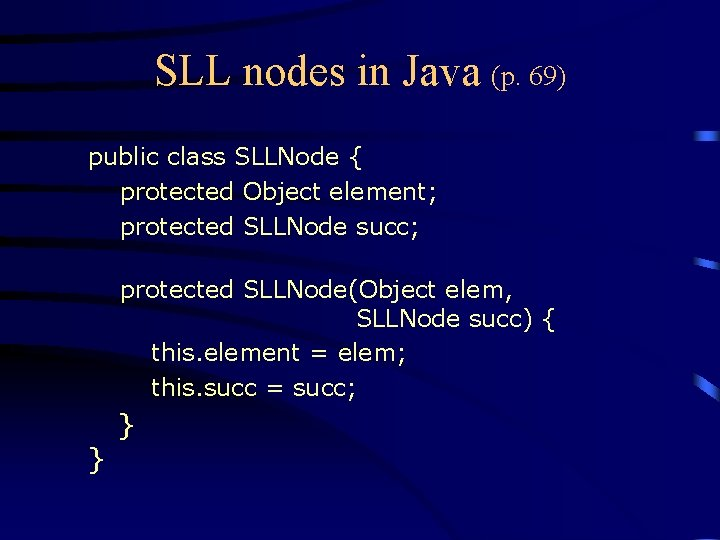SLL nodes in Java (p. 69) public class SLLNode { protected Object element; protected SLLNode succ; protected SLLNode(Object elem, SLLNode succ) { this. element = elem; this. succ = succ; } }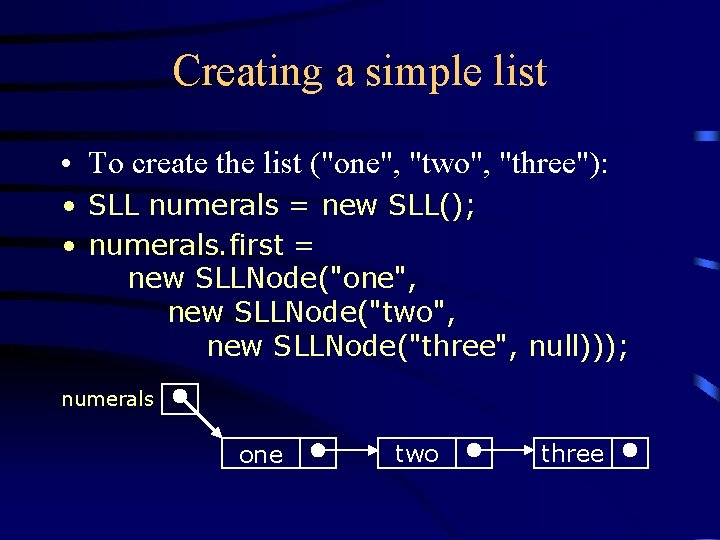Creating a simple list • To create the list ("one", "two", "three"): • SLL numerals = new SLL(); • numerals. first = new SLLNode("one", new SLLNode("two", new SLLNode("three", null))); numerals one two threeTraversing a SLL (p. 70) • The following method traverses a list (and prints its elements): public void print. First. To. Last() { for (SLLNode curr = first; curr != null; curr = curr. succ) { System. out. print(curr. element + " "); } } • You would write this as an instance method of the SLL class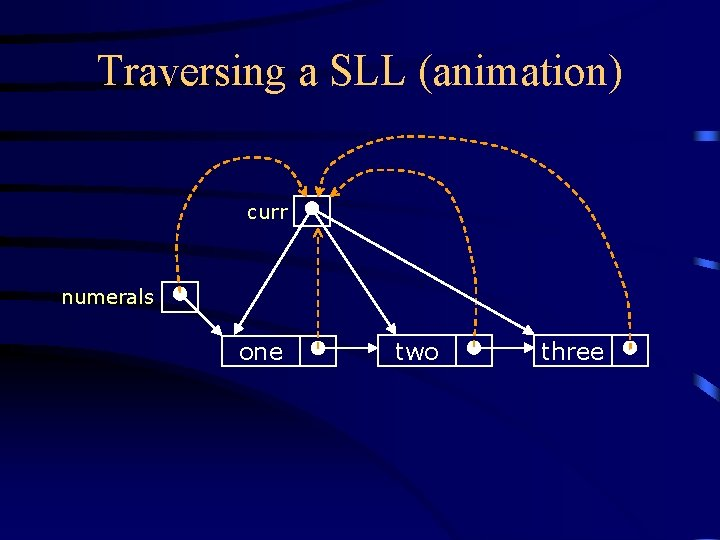Traversing a SLL (animation) curr numerals one two three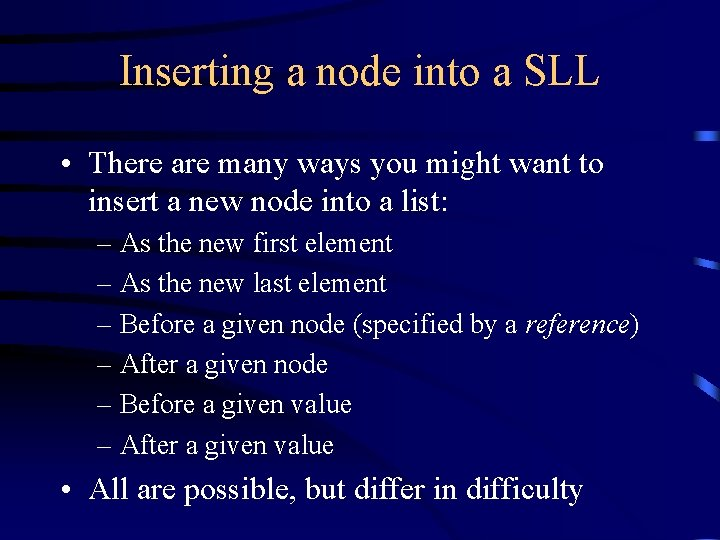Inserting a node into a SLL • There are many ways you might want to insert a new node into a list: – As the new first element – As the new last element – Before a given node (specified by a reference) – After a given node – Before a given value – After a given value • All are possible, but differ in difficulty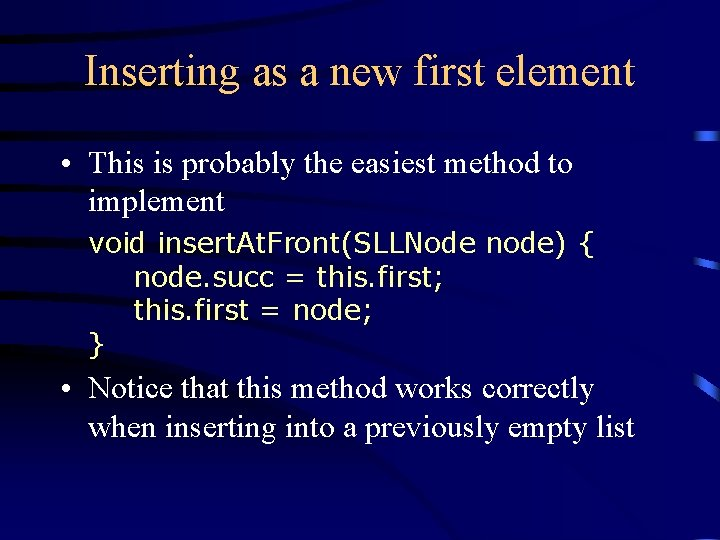Inserting as a new first element • This is probably the easiest method to implement void insert. At. Front(SLLNode node) { node. succ = this. first; this. first = node; } • Notice that this method works correctly when inserting into a previously empty list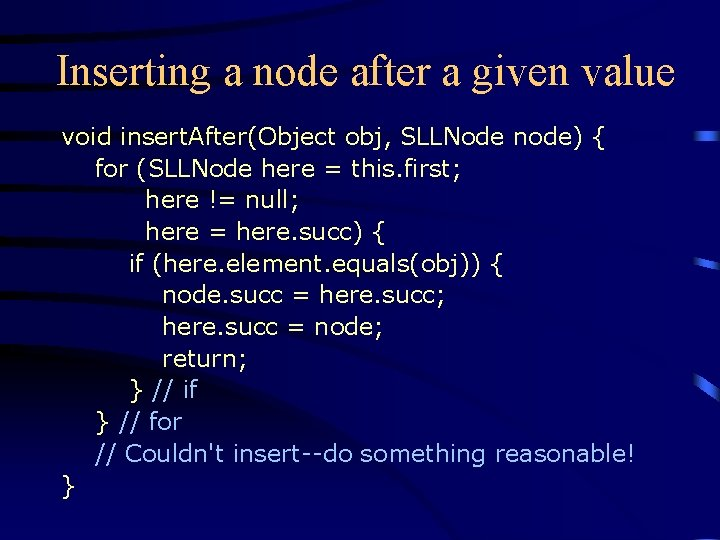Inserting a node after a given value void insert. After(Object obj, SLLNode node) { for (SLLNode here = this. first; here != null; here = here. succ) { if (here. element. equals(obj)) { node. succ = here. succ; here. succ = node; return; } // if } // for // Couldn't insert--do something reasonable! }Inserting after (animation) node 2. 5 numerals one two three Find the node you want to insert after First, copy the link from the node that's already in the list Then, change the link in the node that's already in the listDeleting a node from a SLL • In order to delete a node from a SLL, you have to change the link in its predecessor • This is slightly tricky, because you can’t follow a pointer backwards • Deleting the first node in a list is a special case, because the node’s predecessor is the list header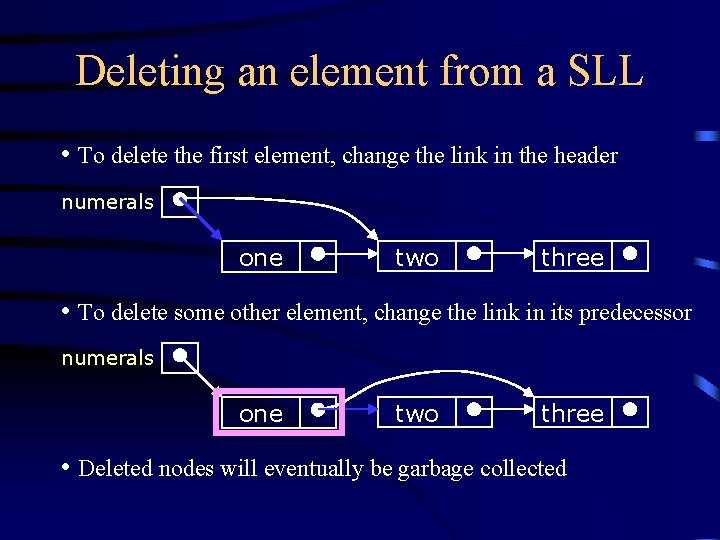Deleting an element from a SLL • To delete the first element, change the link in the header numerals one two three • To delete some other element, change the link in its predecessor numerals one two three • Deleted nodes will eventually be garbage collectedDeleting from a SLL (p. 84) public void delete(SLLNode del) { SLLNode succ = del. succ; // If del is first node, change link in header if (del == first) first = succ; else { // find predecessor and change its link SLLNode pred = first; while (pred. succ != del) pred = pred. succ; pred. succ = succ; } }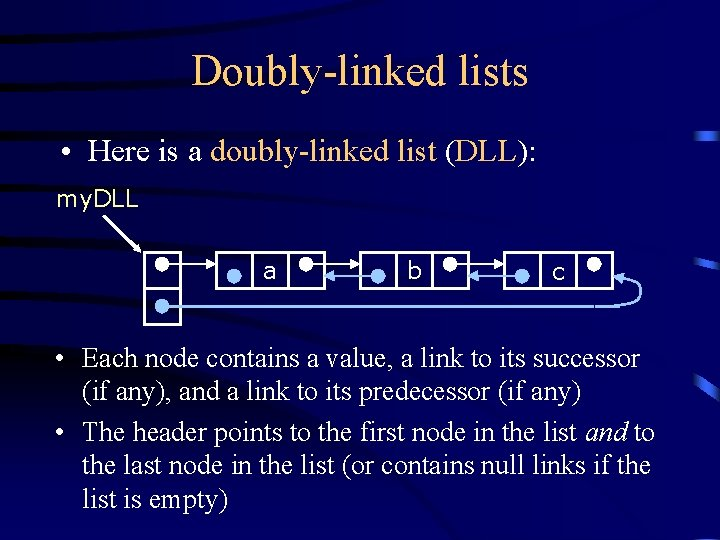Doubly-linked lists • Here is a doubly-linked list (DLL): my. DLL a b c • Each node contains a value, a link to its successor (if any), and a link to its predecessor (if any) • The header points to the first node in the list and to the last node in the list (or contains null links if the list is empty)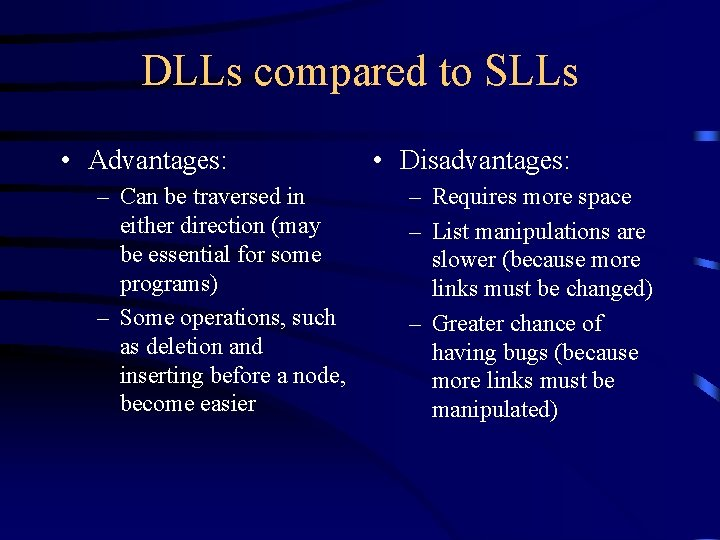DLLs compared to SLLs • Advantages: – Can be traversed in either direction (may be essential for some programs) – Some operations, such as deletion and inserting before a node, become easier • Disadvantages: – Requires more space – List manipulations are slower (because more links must be changed) – Greater chance of having bugs (because more links must be manipulated)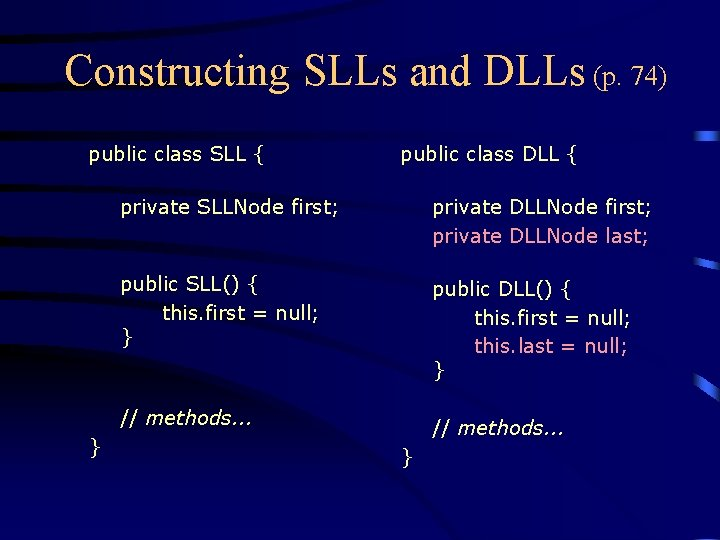Constructing SLLs and DLLs (p. 74) public class SLL { } public class DLL { private SLLNode first; private DLLNode first; private DLLNode last; public SLL() { this. first = null; } public DLL() { this. first = null; this. last = null; } // methods. . . }DLL nodes in Java (p. 75) public class DLLNode { protected Object element; protected DLLNode pred, succ; protected DLLNode(Object elem, DLLNode pred, DLLNode succ) { this. element = elem; this. pred = pred; this. succ = succ; } }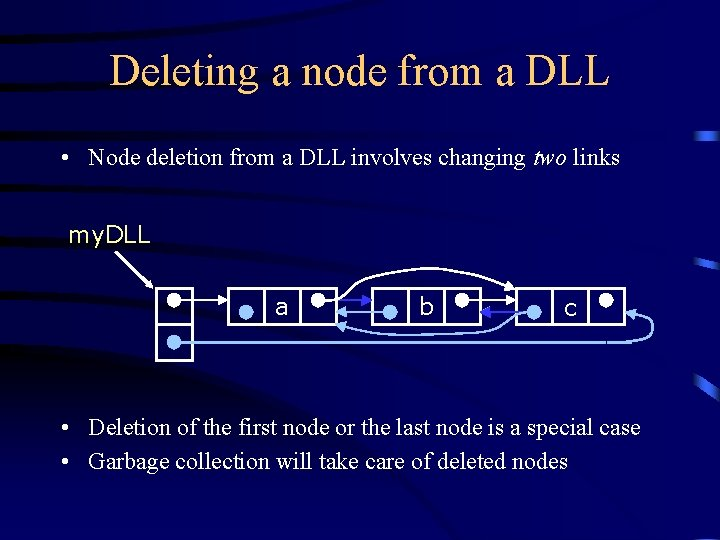Deleting a node from a DLL • Node deletion from a DLL involves changing two links my. DLL a b c • Deletion of the first node or the last node is a special case • Garbage collection will take care of deleted nodesOther operations on linked lists • Most “algorithms” on linked lists—such as insertion, deletion, and searching—are pretty obvious; you just need to be careful • Sorting a linked list is just messy, since you can’t directly access the nth element—you have to count your way through a lot of other elementsThe End